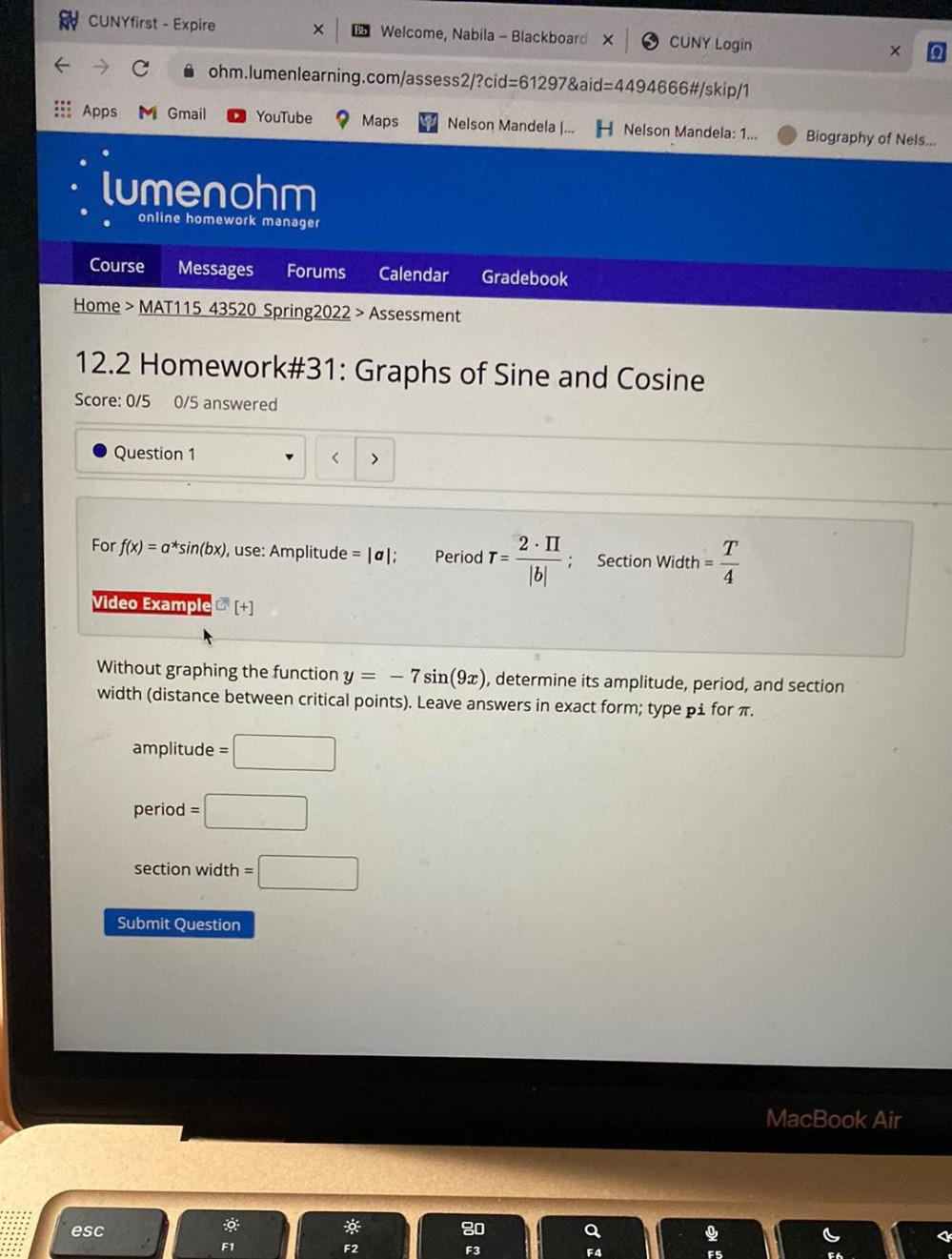Question:

# For f(x) = a*sin(bx), use: Amplitude = |a|; Period T

Last updated: 8/1/2022For f(x) = a*sin(bx), use: Amplitude = |a|; Period T =2.π/|b| ;Section Width =T/4 Without graphing the function y = - 7 sin(9x), determine its amplitude, period, and section width (distance between critical points). Leave answers in exact form; type pi for π. amplitude =? period=? section width=?